# In conversation with Trachette Jackson

Oncology, the study of cancer, is just one of many specialisms which increasingly employs the predictive power of applied mathematics. This issue we chat with Trachette Jackson, professor of mathematics at the University of Michigan, to learn about the surprising effectiveness of mathematics in the treatment of cancer, as well as to hear about her own journey into mathematical oncology.

## Modelling in medicine

Trachette starts by bringing us up to date on how mathematics has been used in cancer treatment. “The mathematical approach has been applied to just about every aspect of tumour growth, starting decades ago.” One aspect is cancer therapeutics: “We write down equations that describe the mechanism of action of new drugs and how the tumour responds, then make predictions about how best to deliver those drugs.” Another aspect is more fundamentally biological, such as how cells are transformed: “You can find mathematical equations about the probabilities of acquiring mutations and under what circumstances a tumour forms, as well as what the composition of that tumour will be and how many cells in that tumour will have these different mutations.” This sort of modelling allows us to diagnose or assess the risk of cancer developing, as well as treat it. Continue reading# How to cheat at cards

As a child I didn’t want to be a mathematician—I didn’t even know that was a job. Instead, for a short time, my dream was to be a crime-fighting card cheat. My interest arose from the TV series Sword of Justice where the main character obtained some help from card cheats in his quest to take down the despicable crime syndicate that had framed him and sent him to prison. (The opening credits on YouTube will attest to the programme’s brilliance.)

Despite the incompatibility of combining crime-fighting and card cheating I told my family that that was to be my chosen career. Unfortunately, I wasn’t very good at it, usually announcing my intentions to cheat before offering to play a quick game with someone. Nonetheless, to this day my family refuse to play cards with me—even Uno.

Along the way, I did learn a thing or two though. So allow me to explain to you an impressive trick that all the best card cheats know and that has some interesting mathematics hidden behind it.

## The perfect shuffle

Every card cheat needs to be able to give the appearance of shuffling a deck of cards so that it remains in order. However, there is an entirely legitimate shuffle that allows us to cheat: the perfect shuffle. It mixes the cards but in a perfectly known and determined way.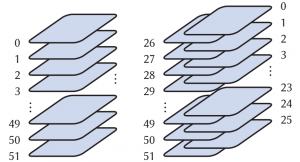A perfect (out) shuffle.

A standard deck has 52 cards. Split them into two equal piles and then interweave the piles so that the new order of cards alternates between the two piles.

The effect on the deck is shown on the right, where the cards are labelled 0 to 51 for reasons which will become clear later.

Instructions on how to do the shuffle are given below. Admittedly, it is not easy and plenty of practice is required but is satisfying once learned and has an impressive ‘wow!’ reaction when performed.

## Eight perfect shuffles

What can we do with the perfect shuffle when we have learned it? First we have an interesting—and hopefully surprising—fact:

Perfect shuffle a deck eight times. It is now back in the order you started with!Modular arithmetic is also used by clocks: they count the time mod 12.

With this you can perform a surprising trick. Put the deck in new deck order. If you perfect shuffle it five times, then the deck looks shuffled. There are patterns if one looks closely but you can show it to someone and with a straight face claim it is mixed up. Do the perfect shuffle three times for them—so now you have done it eight times in total—and to your spectator’s bafflement the deck is suddenly in order!

How can we use mathematics to prove this surprising fact? We start by numbering our cards. As above, from top to bottom, we shall number them 0 to 51, rather than the usual 1 to 52.

Next we use modular arithmetic. Arithmetic modulo $n$ is where we consider all numbers that have the same remainder after division by $n$ to be equivalent numbers. So $5=17 \mod 4$ means that 5 and 17 are equivalent modulo 4 as they both have remainder 1 after division by 4. Sometimes people write $5\equiv 17 \mod 4$ rather than using the equals sign.

With our 0 to 51 numbering the formula for finding where a card goes to after a perfect shuffle is relatively easy:

(Except for card 51) the card at position $x$ goes to position $2x \mod 51$.

For example, card 7 goes to $2 \times 7 \mod 51 = 14 \mod 51$, ie position 14. Note that this is the 15th physical card in the deck as we started counting at 0.

Similarly card 35 goes to \begin{align*} 2 \times 35 \mod 51 &= 70 \mod 51 \\ &= 51 + 19 \mod 51\\ &= 19. \end{align*} That is, card 35 goes to position 19.

Note that the card 7 gets lower in the deck and card 35 gets higher. So immediately we see that a perfect shuffle is beginning to mix the cards.

The formula has the exception of card 51. It is easy to see in the picture on the previous page that this card does not move and so we don’t have to worry about it in the formula—we will ignore it from now on.

But why does this $x\mapsto 2x \mod 51$ result hold? Consider first the top half of the deck, ie all the cards in positions 0 to 25. During the shuffle, the two cards in a pair of consecutive cards get a card between them. So the new positions of the top half cards will be multiplied by 2. Since we can have at most $2\times 25=50$, which is less than 51, we get $x\mapsto 2x\mod 51$ since $2x$ is in this case actually equal to $2x\mod 51$ (rather than just congruent).

For the bottom half cards we see that their positions should also be multiplied by 2 since they get cards between them. However, we note that card 26 (the top card of the bottom half of the deck) needs to go to position 1 (the second physical card position!). One way to do this is to take mod 51 (since $2\times 26 \mod 51 = 1$). It is easy to check that we can use this for all the other cards.

Now let’s see what happens when we do eight perfect shuffles on a particular card, say card 12. We just repeat the process that the card in position $x$ will go to position $2x \mod 51$: $\begin{array}{lccrcr} {\text{once}} & 12 & \to & 24 \mod 51 & & \\ {\text{twice}} & 24 & \to & 48 \mod 51 & & \\ {\text{three times}} & 48 & \to & 96 \mod 51 & =& 45\mod 51 \\ {\text{four times}} & 45 & \to & 90 \mod 51 & =& 39\mod 51 \\ {\text{five times}} & 39 & \to & 78 \mod 51 & =& 27\mod 51 \\ {\text{six times}} & 27 & \to & 54 \mod 51 &=& 3 \mod 51 \\ {\text{seven times}} & 3 & \to & 6 \mod 51 & & \\ {\text{eight times}} & 6 & \to & 12 \mod 51 &=& 12 \mod 51. \end{array}$ Of course, that doesn’t prove that this happens for the whole deck. I may have been cheating by picking one that worked—and you should never trust a card cheat! So let’s do the calculation for a general $x$. If we repeat the $2x\mod 51$ operation we get $2(2x)\mod 51$. Continuing in this way for the full 8 times we see card $x$ goes to position $2^8x\mod 51$. Simplifying mod 51 we get \begin{align*} 2^8 x \mod 51 &= 256 x \mod 51 \\ &=\left( (5\times 51) x + x \right) \mod 51\\ &=\left( 0 + x \right) \mod 51\\ &=x. \end{align*}

Hence, we have proved that eight shuffles move the card in position $x$ back to position $x$. If we have a different number of cards in our deck, then the number of perfect shuffles needed to return the deck to its original order varies. For example, a deck with 50 cards needs 21 perfect shuffles. The number of shuffles required for the deck is called the order. The following table shows the order of the perfect shuffle for a selection of decks where $N$ denotes the number of cards:

$N$ order
4 2
6 4
8 3
10 6
12 10
14 12
16 4
18 8
50 21
52 8
54 52

The word order arises due to a connection with group theory and the order of elements of a certain group. The details of this and many more hardcore mathematical facts can be found in Magic Tricks, Card Shuffling and Dynamic Computer Memories by S Brent Morris: one of its appendices is a list of all orders for $N$ up to 200.

## Binary fun with in and out perfect shuffles

Up to this point I have talked of a perfect shuffle, ie singular. In fact, there are two types:

• A perfect out shuffle: the top card remains the top card.
• A perfect in shuffle: the top card becomes the second card.A perfect in shuffle.

The perfect out shuffle is just what have been calling the perfect shuffle. The in shuffle is the new one. Its effect on deck order can be seen below—the top card ends up ‘in’ the deck.

By combining out and in shuffles we can move the top card to anywhere in the deck. And, as we shall see, from a mathematical perspective we get a surprising appearance of binary.

Alex Elmsley (1929–2006) was a mathematician and magician. He studied physics and mathematics at the University of Cambridge before becoming interested in computers. Among magicians he is known for inventing a convincing false count of cards which is now known as the Elmsley Count.

Elmsley was very interested in the perfect shuffle (which among magicians is also known as the Faro shuffle) and investigated the mathematics behind it. In particular, he found a method for moving the top card to any position in the deck through a sequence of in and out shuffles.

Let us suppose we wish to move the top card to position $k$ in the deck. (We keep the numbering of the cards from 0 to 51 so the top card is in position 0.) To move the card via a sequence of in and out shuffles we proceed as follows:

Translate $k$ to binary. Then do out and in shuffles where $\text{out} = 0$ and $\text{in} = 1$.

Note how brilliant it is that ‘o’ looks like 0 and ‘i’ looks like 1.

For example, suppose we want to move the top card to position 13. Well, 13 is written as 1101 in binary. So we do ‘i i o i’: in in out in.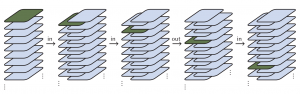Moving the top card to position 13.

So why does this work? Imagine we have a card in position $x$ in the top half of the deck. (The case of the bottom half is similar and is, of course, left as an exercise.) What does an out shuffle do to the binary representation of the number? Since an out shuffle is $x\mapsto 2x \mod 51$ it just adds a zero to the representation. So, a card at position 101 moves to 1010. This is no different to when we tell children that to multiply by 10 they can just place a 0 at the end.

Now, an in shuffle is given by $x\mapsto 2x +1 \mod 52 .$ That is, multiply by 2, add 1 and take mod 52 (note, not 51 this time). This can be checked in the same way as for the out shuffle. For a binary representation this will just add a 1 to the end. Thus, the card in position 101 moves to position 1011.

To put this all together, consider what happens in the above example where we move the top card to position 13. That is, we apply in in out in and track the progress of the initial top card. The first in shuffle moves the top card to position 1 in the deck. The second in shuffle moves it to 11. The out moves it to 110 and the last in moves it to position 1101.

OK, so this is an unexpected and charming appearance of binary but it only moves one card. That’s useful, but let’s see how we can move more cards and cheat in a real game.

## Dealing four aces

An impressive bit of cheating is to deal yourself four aces in a game of poker—that’s a hand that is hard to beat. For our version we need a game with three other players. Take out the four aces and place them on top. A card cheat can do this secretly with ease. Now, do two perfect out shuffles. Deal the cards starting with you, ie go round in a circle giving each person a card until everyone has five cards each. You now have a hand with the four aces!

The reason that this works is simple. At the start, the pack (starting at the top) looks like

AAAAxxxxxxx…

where A denotes an ace and x denotes an indifferent card (whose value is unimportant).Two perfect out shuffles give you all the aces (green).

Do the first shuffle and indifferent cards get placed between the aces. So we get

AxAxAxAxxxxxxx…

Do the second shuffle and we get a set up ready for a good hand when there are three other players:

AxxxAxxxAxxxAxxxxxxx…

Well, of course if you are going to cheat and win all the time, people are going to notice. To dodge this professional card cheaters would often work in pairs and the winning would alternate between the two cheaters, reducing the chances of discovery. So in this poker game of four players you need an accomplice. Suppose you are sitting in a circle with you dealing first to the person on your left and then going clockwise.AAAA!

Wherever your accomplice sits you will be able to deal them the four aces. As before arrange the four aces on top. Suppose your partner is sitting at position 3. You need to deal them the third card—the card at position 2 in our numbering. Let’s move that top ace to position 2. In binary the number 2 is 10 so we do ‘io’ perfect shuffles. Hey presto, the top ace is now in the right place and furthermore so are the other aces as the two shuffles put three cards between each of them. A normal deal now will give your partner-in-crime all four aces. Let’s see that in a diagram:

start: AAAAxxxxxxx…
in shuffle: xAxAxAxAxxxxx…
out shuffle: xxAxxxAxxxAxxxAxxxxx…

Wherever your partner sits you can do the right sequence of in and out shuffles to ensure that they receive the aces!

If you wish to know more about card shuffling and mathematics, then the best book to read is (as mentioned already) Magic Tricks, Card Shuffling and Dynamic Computer Memories by S Brent Morris. And remember, card cheating is a crime—so don’t get caught!

## How to do the perfect shuffleSplitting the deck. Image: C Houston

The perfect shuffle requires cards in good condition so buy a new deck on which to learn and don’t use it for anything else—cards buckled in card games will not weave very well. There are various methods to achieve a perfect shuffle. This version is done ‘in the hands’ but some can be done on the table and don’t look in any way suspicious.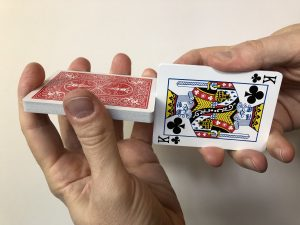Squaring the cards. Image: C Houston

Hold the lower half of the deck in your left hand with fingers arranged around as above. The right hand has a similar grip but does not go all the way round and the index finger sometimes contacts the top of the deck, sometimes not.

Butt the cards together to square them up. If the cards are not square, then perfect shuffling is impossible. Press the halves together, with the right index finger touching both top cards so that the halves can be put in position. For an out shuffle the right hand part should be slightly higher so that the left hand top card will go under the right hand top card at the end of the shuffle. For an in shuffle the right hand part needs to be lower.A perfect shuffle. Image: C Houston

Remove the index finger and begin applying pressure at the bottom corner where the two packs meet. Once interweaving has started let the deck ‘do the work’. It took me many weeks to be able to do this consistently so don’t be discouraged if you can’t do it straight away. Like many skills it needs practice!Would you like to have a mathematical object named after yourself? You no longer need to be Pythagoras to eternalise yourself in the terminology of mathematics.

#### A polyhedron is not just for ChristmasThis is Ecki. He lives in Polytopia.

Mathematicians currently know over a hundred trillion  different kinds of polyhedron and only a very small percentage of them actually have a name, like cube or pyramid. Not only does the cube possess a name and fame, it is also realised in gigantillions of models all over the world. But not for most polyhedra. These helpless shapes are nameless, unrealised, and lost in abstraction. You can help change that, today.

## Who are we?

Polytopia is a foster home for all polyhedra with up to nine vertices. We give them a home, regardless of their prominence or whether they’ve already got a name. When visiting Polytopia, you’ll be able to see all the polyhedra who have already found loving homes and, more importantly, meet the ones still waiting to be adopted.

#### But what can you do?

You can help us make a change and adopt one of these little geometrical creatures. By giving them a name and building a physical model of them, you can bring them into the real world. Visit us at Polytopia today and be inspired to rescue your new best friend from the realm of abstraction.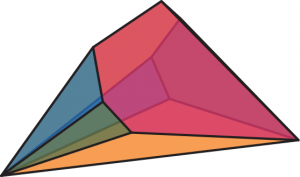One of the polyhedra that lives in Polytopia.

There are several definitions of polyhedra in mathematics. Let us start with talking about polygons, which you are probably already familiar with. A polygon is a two-dimensional shape, consisting of straight line segments, called edges, and points, called vertices, where two edges meet. The simplest example of a polygon is a triangle. One important type of polygon is the regular polygon: those which have edges of the same length, and with all internal angles the same. Examples of regular polygons are the equilateral triangle and the square.“I named my polyhedron Seems to be Piez and had a lot of fun crafting it.”“My polyhedron is called Oktavius and makes a lovely decoration for my room.”

From polygons we can naturally build up to polyhedra. A polyhedron is a geometrical object bounded by a finite number of polygons. In other words, a polyhedron is a three-dimensional object, whose faces are polygons, whose edges are the straight line segments where two such faces meet, and whose vertices are points where three or more faces meet.

One of the simplest (and most famous) polyhedra is the cube. It is built by glueing squares together and it consists of eight vertices connected by twelve edges and six faces.

In Polytopia, we are only interested in convex polyhedra, which means that all inner angles between two edges or two faces are less or equal to 180°. Thus, no indentations, holes or cavities are allowed, and all vertices and edges are ‘pointing outwards’.

The superstars of the polyhedral world are the Platonic solids. These five polyhedra, each consisting only of identical regular polygons, are named after the ancient Greek philosopher Plato. Starting with regular triangles, we can use them to build three of the five Platonic solids: the tetrahedron, the octahedron, and the icosahedron. Do you already know the two remaining Platonic solids? (Hint: one of them is the cube.)Three Platonic solids. Who’s missing?

Now you might ask yourself: how do we differentiate the polyhedra?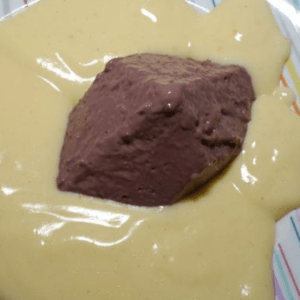“It was so much fun modelling my polyhedron Pullyeder out of chocolate ice cream, especially because I ate it afterwards!”“Since I was in need of a new pillow, I decided to sew my polyhedron Flori into one.”

We at Polytopia distinguish polyhedra by their combinatorial type and not their geometric realisation. The combinatorial type describes the structure of the vertices, edges, and faces of a polyhedron but it is not sensitive to the size or even the shape of a polyhedron. Given two polyhedra, take out the faces as if they were made of glass. What remains is the frame of the edges. Now imagine that you can mould and transform this frame in any way you like, but you cannot disconnect any of the edges or vertices. If you can transform the frame of one polyhedron into the other without making or breaking any connections, then these two polyhedra are considered combinatorially equivalent.

The two pictures on the next page show the cube but in different geometric realisations. The one on the left looks like what you might expect and the one on the right was generated by modifying the size and shape of the usual cube. But notice that the vertices can still be matched to one another, preserving the edges’ connections. Therefore, they are combinatorially equivalent, and are both called Cube.Cube standing up straight, and Cube slouching.

Unfortunately, even with combinatorial equivalence, there are so many different polyhedra that it is infeasible for mathematicians to name them all. That’s why we here at Polytopia invite you to name as many polyhedra as you can. Every little helps.

 114,683,158,574,357 to be precise. This is the number of combinatorial types of polyhedron with up to 18 vertices. No mathematician has been able to give the number for 19 vertices exactly: so far for more than 18 vertices, only upper bounds on the number of combinatorial types are known. Even the computers we have today would take forever to construct and count all types for 19 vertices.# Automated joke generation

You wouldn’t expect a computer to be able to make up a funny joke—it seems like a uniquely human ability. In order for a computer to understand the jokes that it is making, it would need to have deep semantic understanding of words, which is infeasible. And it can be difficult to explain why a joke is funny.

However, it is feasible to use factor graphs to define a relatively simple model for what makes a specific class of jokes funny. A computer can then apply this methodology to make funny jokes, although it will not understand them.

Mathematicians can use factor graphs to represent the factorisation of a function. As an example, suppose we have the function

\begin{equation*} f(X_1, X_2, X_3) = X_1 (X_1 + X_2 + X_3). \end{equation*}

Then $f$ can be factorised as \begin{equation*} f(X_1, X_2, X_3) = g(X_1) h(X_1, X_2, X_3), \end{equation*} where $g(X_1) = X_1$ and $h(X_1, X_2, X_3) = X_1 + X_2 + X_3$. ​ The factor graph for $f$ is: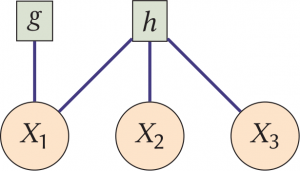It shows how $f$ can be factorised, and which variables appear in each factor. To make the graph, we label the factors in square boxes, the variables in circular boxes, and connect a variable to a factor if that variable is a term in the factor.

Factor graphs are useful for representing the factorisation of probabilities and they aid in visualising and piecing together the model’s connections. As another example, the combined probability that there will be rain on Monday and Tuesday ($R_M$ and $R_T$) can be factorised as

\begin{align*} P(R_M, R_T) &= P(R_M) P(R_T \mid R_M) \\ &= f_1(R_M) f_2(R_M, R_T). \end{align*}

Factor $f_1$ determines whether it rains on Monday, and factor $f_2$ determines if it rains on Tuesday given that it rained on Monday. The factor graph is:Unsupervised joke generation from big data, a paper by Saša Petrović and David Matthews from 2013, is one of the most cited papers on automated joke generation. It uses factor graphs to generate jokes of the form

I like my $X$ like I like my $Y$: $\boldsymbol{Z}$.

For example:

I like my men like I like my coffee: hot.
I like my secret like I like my subset of $\mathbb{R}^2$: open.

The authors assumed that the probability that a joke of this form is funny is a function of its inputs, $(X, Y, Z)$. Technically the authors created a model for the probability that you would randomly choose the triple $(X, Y, Z)$ when choosing from a population of funny jokes. But we are interested in choosing triples that are likely to be funny. Fortunately, Bayes’ theorem tells us that the two quantities are proportional to each other. If $A$ and $B$ are random events, Bayes’ theorem says \begin{equation*} P(A \mid B) \propto P(B \mid A) P(A). \end{equation*}

We now let $A$ be the event that we choose the triple $(X, Y, Z)$, and let $B$ be the event that we choose a funny triple; so if a particular triple $(X, Y, Z)$ is more likely to be chosen by the model then its associated joke is more likely to be funny. Petrović and Matthews further assumed that $P(A \mid B)$ can be factorised using the factor graph below, which I will now go through.## Factor 1: $\text{Co-oc}_{X}, \text{Co-oc}_{Y}$

A joke of the form ‘I like my $X$ like I like my $Y$: $Z$’ will only make sense if the adjective $Z$ is often used to describe $X$ and $Y$. Without this assumption we would get anti-jokes such as

I like my goat like I like my Ferris wheel: intentional.

The factor $\text{Co-oc}_X$ is a measure of the co-occurrence. If we are given non-random values $x, z$ then we can evaluate:

\begin{equation*} \text{Co-oc}_X(x, z) = \frac{f(x, z)}{\sum_{x^\prime, z^\prime} f(x^\prime, z^\prime)}, \end{equation*}

where $f(x, z)$ counts the number of times that adjective $z$ is used to describe $x$ in a large corpus (a collection of written works). Petrović and Matthews used the Google Ngram data set as their corpus. We divide $f(x, z)$ by the sum of $f(x^\prime, z^\prime)$ over all pairs $(x^\prime, z^\prime)$ in order to scale $\text{Co-oc}_X(x, z)$ to be in the interval $[0, 1]$, like a probability (it will be used like a probability in Factor 2). $\text{Co-oc}_Y$ is just the same but with $y$ replacing $x$. ​

## Factor 2: $\text{Diff}(X, Y)$

‘I like my $X$ like I like my $Y$: $Z$’ will only work as a joke if $X$ and $Y$ are quite different nouns. Without this assumption we would get anti-jokes such as:

I like my tea like I like my coffee: hot.

So the authors wanted to give a higher score to dissimilar nouns:

\begin{equation*} \text{Diff}(x, y) = \frac{1}{\textrm{similarity}(x, y)}, \end{equation*}

where the similarity function is estimated from the corpus. We set the probability of seeing the (noun, adjective) pair $(x, z)$ to be $p(x, z) = \text{Co-oc}_X(x, z)$, and then define

\begin{equation*} p(x) = \frac{f(x)}{\sum_{x^\prime} f(x^\prime)}, \end{equation*}

where $f(x)$ counts the number of occurrences of $x$ in the corpus. So $p(x)$ is the probability that we would choose $x$ if we chose a random word from the corpus. We also define

\begin{equation*} p(z \mid x) = \frac{p(x, z)}{p(x)}, \end{equation*}

so $p(z \mid x)$ is the probability that you have chosen adjective $z$, given that you chose a random (noun, adjective) pair from the data set and you see that you have chosen noun $x$. We define $p(y)$ and $p(z \mid y)$ similarly. The authors then chose a similarity function that resembles a correlation function between $X$ and $Y$:

\begin{equation*} \textrm{similarity}(x, y) = \frac{\sum_z p(z \mid x) p(z \mid y)}{\sqrt{\sum_z p(z \mid x)^2 \times \sum_z p(z \mid y)^2}}. \end{equation*}

If $x = y$ then $\textrm{similarity}(x, y) = 1$, and if $x$ and $y$ are commonly described using the same adjectives then the similarity will be close to $1$. And if $x$ and $y$ are very rarely described using the same adjectives then $\textrm{similarity}(x, y)$ will be close to $0$. ​

## Factors 3 & 4: $\text{Rare}(Z)$ & $\text{Sens}(Z)$

The third factor encodes that the joke is funnier if $Z$ is not a common adjective. Without this assumption we would get anti-jokes such as:

I like my dogs like I like my quality of life: good.

The third factor is just defined as the reciprocal of the frequency of adjective $z$:
\begin{equation*} \text{Rare}(z) = \frac{1}{f(z)}.\end{equation*}The final factor encodes that the joke is funnier if $Z$ has many different senses. ‘I like my $X$ like I like my $Y$: $Z$’ jokes are often funny because one meaning of $Z$ is used for $X$ and another is used for $Y$. Without this assumption we would get anti-jokes such as:

I like my rectangles like I like my parallelograms: quadrilateral.

Just because $Z$ is chosen to have multiple senses doesn’t guarantee that it will be used in different senses for $X$ and $Y$: it is just an approximation. The final factor is

\begin{equation*} \text{Sens}(z) = \textrm{senses}(z), \end{equation*}

where $\textrm{senses}(z)$ is derived from the Wordnet corpus. ​ The probability that the authors chose a particular triple $(x, y, z)$ is then given by

\begin{equation*} \frac{\text{Co-oc}_X(x, z) \text{Co-oc}_Y(y, z) \text{Diff}(x, y) \text{Rare}(z) \text{Sens}(z)}{\sum_{x^\prime, y^\prime, z^\prime} \text{Co-oc}_X(x^\prime, z^\prime) \text{Co-oc}_Y(y^\prime, z^\prime) \text{Diff}(x^\prime, y^\prime) \text{Rare}(z^\prime) \text{Sens}(z^\prime)}, \end{equation*}

where we divide by the sum of all possible triples in order to make sure that the probabilities all sum to $1$. It was too expensive to compute the sum over all possible triples $(x, y, z)$ so the authors restricted themselves to some selected values of $x$, and for each of these they computed the conditional probability of $(X, Y, Z)$ given that $X = x$, which is computationally feasible. ​ The authors tested their model, comparing the jokes that they generated to a random selection of jokes found on Twitter. A small panel judged that their method can generate funny jokes: 16.3% of the jokes generated by their model were found funny, compared to 33.1% for the Twitter jokes. Here are two of the jokes generated by their model:

I like my coffee like I like my war: cold.
I like my relationships like I like my source: open.

In summary, factor graphs can be used to represent probability models, and these can also be used to generate jokes! Why not have a go at making your own jokes using these principles—Chalkdust would love to hear from you, especially if you come up with a good mathematical joke.# Kepler’s barrels

Kepler went out shopping for a barrel full of wine,
He had a problem with the price the merchant would assign,
The merchant used a measure stick to calculate the price,
Into the barrel it did drop,
Through a hole at centre top,
And down and left until it stopped,
He thought it imprecise.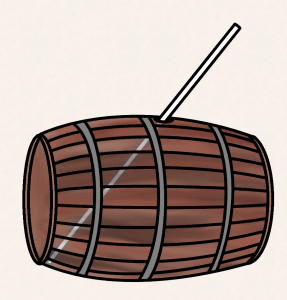He said there’d be a certain price for a barrel short and fat,
And another that was tall and thin would cost the same as that,
But while the short fat barrel would hold lots and lots of wine,
The tall thin one would hold much less,
This observation caused distress,
Kepler started to obsess,
He didn’t think it fine.Though Kepler paid the merchant then, he never could forget,
That inconsistent pricing, well, it really made him fret,
Day after day he pondered as he went about his work,
The fairness of the merchant’s rate,
He could no more procrastinate,
Or he would go berserk.For any given pricing, how much wine could one consume?
What measurement of barrel gave the maximum volume?
He noted that a cylinder was almost the same shape,
As the barrel he’d been using,
For his celebratory boozing,
And so he began his musing,
He could approximate.

For cylinders of different heights he worked out the diameter,
Assuming that the merchant’s measure was a fixed parameter,
Trying different values gave a groundbreaking conclusion,
Diameter, times by root 2,
Would give the perfect height value,
A maxed-out volume would ensue,
It was no illusion.Then Kepler found out something new that really made him cheer,
All of Austria’s barrels had this ratio, or near,
So the barrel that he’d purchased had a fair price after all,
A few might slightly deviate,
But Kepler could appreciate,
The volume change that this creates,
Is imperceptible.But the story doesn’t end here, because Kepler’s observation,
That the points around the max have but a tiny deviation,
Inspired Fermat’s theorem about stationary points,
It might not seem so obvious,
But this led on to calculus,
A field meritorious,
That never disappoints.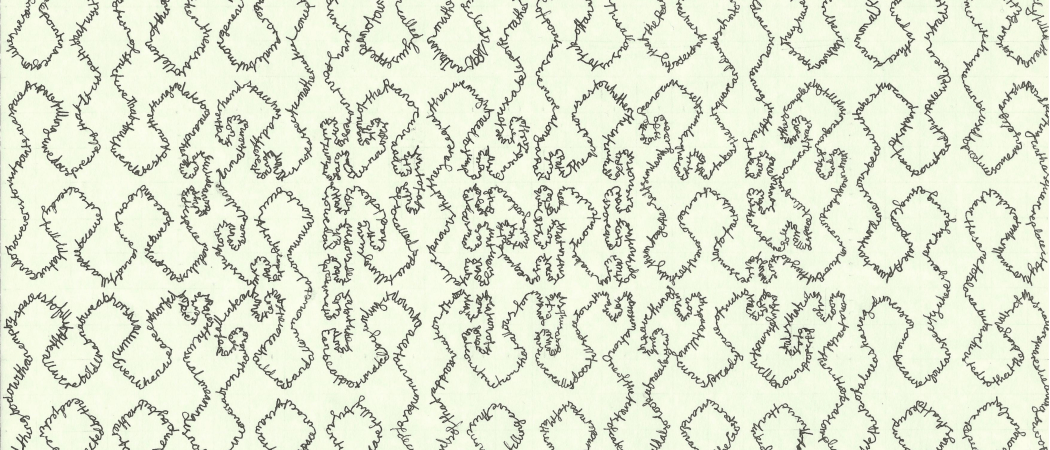# What can you do with this space?

A few years back, the students and staff at my school were set an art challenge over the summer break. Those who chose to take part were given a blank postcard and asked the question: What can you do with this space?

I feel that the obvious answer to this question is ‘fill it’. But in mathematics, the combination of the words space and fill links to a very specific concept: space-filling curves. In this article, I’m going to explain what these are, and how this leads to my entry (shown above).

## Space-filling curvesA detail of my postcard.

There are many variations on the theme of space-filling curves, but the original and simplest version is a continuous curve that passes through every point in the unit square. Formally, it is a continuous surjective function $\gamma \colon [0,1] \to [0,1]^2$. Note that it only needs to be continuous and doesn’t have to be differentiable, so sharp corners are allowed.

The first space-filling curve was designed by Peano in 1890. Surprisingly, his paper has no pictures. A year later, based on Peano’s work, Hilbert came up with a slightly different construction and included pictures. Hilbert curves seem to be the more popular of the two, with about 20 times more search hits on the internet. You might conclude that pictures are important in mathematics; I couldn’t possibly comment. Continue reading# Weirdonacci numbers

We all know how the Fibonacci numbers, $F_n$, work: each number in the sequence is the sum of the previous two numbers, and if we make the usual start, we get $\begin{array}{cccccccc} F_1 & F_2 & F_3 & F_4 & F_5 & F_6 & F_7 & \cdots \\[1mm] 1 & 1 & 2 & 3 & 5 & 8 & 13 & \cdots \end{array}$ The numbers in this sequence grow larger and larger, and can be expressed with the help of the golden ratio $\phi= (1+\sqrt{5})/2$, by the surprising formula $F_n = \frac{\phi^n-(-\phi)^{-n}}{\sqrt{5}}.$ There is a vast amount of literature about this number, $\phi$, to which this article will make no contribution whatsoever.

Instead, we’re going to think about the consequences of interpreting the rule slightly differently. Since this interpretation of the rules is a little weird, we’ll call these the Weirdonacci numbers, $W_n$. One can imagine that this new sequence comes from someone who has misunderstood the construction of the Fibonacci numbers. Instead of adding up the last two numbers of the sequence, the person would add the last two digits in the sequence.

As a consequence, we are still going to get each term by adding together the previous two numbers, but we will think of 13 as two numbers (digits), 1 and 3. The sequence will now grow differently.

We get: $\begin{array}{ccccccccccccc} W_1 & W_2 & W_3 & W_4 & W_5 & W_6 & W_7 & W_8 & W_9 & W_{10} & W_{11} & W_{12} & \cdots \\[1mm] 1 & 1 & 2 & 3 & 5 & 8 & 1 & 3 & 4 & 7 & 1 & 1 & \cdots \end{array}$

Notice that after 10 terms, the sequence starts repeating itself: we can write this as $W_{\ell+j}=W_j$, where $\ell=10$ is the length of the cycle.

But there is.

Since the digits are what matters here, it is important to notice that this sequence depends on the base in which the numbers are expressed.

## The inevitability of cycles

Let’s see what happens with a couple of other choices of base. We will write $W_n^{(b)}$ for the Weirdonacci numbers generated in base $b$. In base eight, we have the following sequence with cycle length $\ell=7$: $\begin{array}{cccccccccc} W_1^{(8)} & W_2^{(8)} & W_3^{(8)} & W_4^{(8)} & W_5^{(8)} & W_6^{(8)} & W_7^{(8)} & W_8^{(8)} & W_9^{(8)} & \cdots \\[1mm] 1 & 1 & 2 & 3 & 5 & 1 & 0 & 1 & 1 & \cdots \end{array}$

In base nine we have the sequence below with cycle length $\ell=11$: $\begin{array}{cccccccccccc} W_1^{(9)} & W_2^{(9)} & W_3^{(9)} & W_4^{(9)} & W_5^{(9)} & W_6^{(9)} & W_7^{(9)} & W_8^{(9)} & W_9^{(9)} & W_{10}^{(9)} & W_{11}^{(9)} & \cdots \\[1mm] 1 & 1 & 2 & 3 & 5 & 8 & 1 & 4 & 5 & 1 &0& \cdots \end{array}$

which brings us back to 1,1, so we see that in each case the sequence jumps back to the start after some number of terms.

We might be tempted to conjecture that this always happens, but we should also bear in mind that three cases are a rather slender foundation to build a conjecture on. It’s worth looking at a few other possibilities before committing to trying to prove something.

This tempting conjecture would also prove true in base seven (you should use the margins of this page to check this), but in fact fails in base six: $\begin{array}{cccccccc} W_1^{(6)} & W_2^{(6)} & W_3^{(6)} & W_4^{(6)} & W_5^{(6)} & W_6^{(6)} & W_7^{(6)} & \cdots \\[1mm] 1 & 1 & 2 & 3 & 5 & 1 & 2 & \cdots \end{array}$

Here, it has gone into a cycle, but it didn’t return to the start of the sequence. So in every case we still get a cycle, but now we know that the loop might not go back all the way to the beginning. This leads to a reasonable conjecture: no matter which base you choose, the Weirdonacci numbers will eventually go into a cycle and repeat forever.

It isn’t too hard to see that this must be the case (once you think about it the right way).

If we work in base $b$, then there are only $b$ distinct numbers that can occur in the sequence. But if there are only $b$ distinct numbers, there can’t be more than $b^2$ pairs of numbers. Then a sequence of more than $b^2$ terms must have some repeated pairs. Wherever we spot a pair repeating, we have identified a cycle.

So not only do we know that the Weirdonacci numbers always eventually repeat, we know that the length of the cycle can’t be any longer than $b^2$. (Question: can it be as long as $b^2$?)

We can see from the above example that the cycle doesn’t have to start with the pair of numbers 1,1. In fact we can start a Weirdonacci sequence with any pair of numbers we like, and it doesn’t matter what the starting values are, or what base we use, eventually the sequence must enter a cycle. One of the first questions we started thinking about was what the length of a cycle could be.

## Cycles can be any length

We’re going to look at what lengths cycles can be now. The maths gets a bit more complicated, so don’t feel embarrassed about skipping to the conclusions after the scorpions at the end of this section, and coming back to this later.

### Fibonacci numbersLet us write $\mathcal{F}_{n}(\alpha,\beta)$ for the $n$th Fibonacci number, with initial values $\alpha$ and $\beta$. For example, if we start the sequence with 2,5 we obtain $2,5,7,12,\ldots$ and so $\mathcal{F}_3(2,5)=7$ and $\mathcal{F}_4(2,5)=12$.

We can show (this is left as an exercise for the reader) that these new sequences are related to the usual Fibonacci numbers by the formula

$$\mathcal{F}_{n}(\alpha,\beta)=\alpha F_{n-2}+\beta F_{n-1},$$

where the usual Fibonacci sequence had to be a little extended by adding the numbers $F_{-1}=1$ and $F_0=0$. Look at the same example as above (the sequence starting with 2,5), this formula correctly tells us that $\mathcal{F}_4(2,5)=5F_3+2F_2=5\times 2+2\times 1 = 12.$

We easily verify that $\mathcal{F}_{1}(\alpha,\beta)=\alpha$ and $\mathcal{F}_{2}(\alpha,\beta)=\beta$, as well as $\mathcal{F}_{n}(1,1)=F_{n}$.

### Weirdonacci numbers

Let us write $W^{(b)}_n(\alpha,\beta)$ for the $n$th Weirdonacci number, generated in base $b$, and with initial numbers $\alpha$ and $\beta$. As long as $\mathcal{F}_{n}(\alpha,\beta)$ is smaller than the base $b$, the Weirdonacci numbers $W^{(b)}_n(\alpha,\beta)$ are equal to $\mathcal{F}_{n}(\alpha,\beta)$.

We then define as $k$ the smallest integer such that  $\mathcal{F}_{k}(\alpha,\beta)$ < $b$ $\le$ $\mathcal{F}_{k + 1}(\alpha,\beta)$. Therefore for any 1 $\le$ $n$ $\le$ $k$,
$W_n^{(b)}(\alpha,\beta)=\alpha {F}_{n-2}+\beta {F}_{n-1}.$

When we reach a number greater than the base $b$, the next two Weirdonacci are generated: remember that in base 10, $W_5^{(10)}=5$, $W_6^{(10)}=8$ and since $8+5=13$, one writes $W_7^{(10)}=1$ and $W_8^{(10)}=3$. We’ll call this a collapse.

In general, we can write $\mathcal{F}_{k+1}(\alpha,\beta)=qb+r$, so that the next two Weirdonacci numbers are $W^{(b)}_{k+1}(\alpha,\beta)=q$ and $W^{(b)}_{k+2}(\alpha,\beta)=r$. In fact, $q$ must be 1, because otherwise at least one of the two previous Weirdonacci numbers would have to be greater than $b$. Consequently, we can write $r=\mathcal{F}_{k+1}(\alpha,\beta)-b$.

It follows that every loop must at least contain one pair of the form $(1,\beta)$, which it is natural to consider as the start of the cycle. Starting with 1 and $\beta$, we can write
$\begin{array}{ccccccc} W_1^{(b)}(1,\beta) & W_2^{(b)}(1,\beta) & \cdots & W_k^{(b)}(1,\beta) & W_{k+1}^{(b)}(1,\beta) & W_{k+2}^{(b)}(1,\beta) & \cdots \\[1mm] 1 & \beta & \cdots & \mathcal{F}^{(b)}_k(1,\beta) & 1 & r & \cdots \end{array}$
where $r=\mathcal{F}_{k+1}(1,\beta)-b$.

### Simple loops

A loop of length $\ell=k$ would be formed if $r=\beta$. We’ll call loops that arise this way simple loops. Of course this is not the only way to generate a loop, as it may take several collapses for the loop to close, but it makes sense to think about the simplest possibility first.

The equation
$\beta = \mathcal{F}_{k+1}(1,\beta)-b$
gives us a relation between $b$, $\beta$ and $k$ for all simple loops. We can then rewrite this equation as
$b-F_{k-1}=\beta(F_k-1).$
If we pick a desired cycle length $k=\ell$, we can use this formula to find a base and starting numbers to give this cycle length. This means that cycles of every length are possible.

There’s lots more we could think about here, including the following challenge.

Challenge 1:
The Weirdonacci sequences $W_n^{(6)}$ and $W_n^{(10)}$ both have a cycle of length 4.

Show that if the base $b$ is even, then it is possible to pick two starting numbers that lead to a Weirdonacci sequence with a cycle of length 4.

(If you’re stuck, there is a hint at the end of the article.)

### Non-simple loops

Of course, one could try to find equations describing non-simple loops—those with more than one collapse. For example, we could look for loops like this (we’ve left out the $(1,\beta)$s in the top row): $\begin{array}{ccccccccccc} W_1^{(b)} & W_2^{(b)} & \cdots & W_k^{(b)} & W_{k+1}^{(b)} & W_{k+2}^{(b)} & \cdots & W_{k+q}^{(b)} & W_{k+q+1}^{(b)} & W_{k+q+2}^{(b)} & \cdots \\[1mm] 1 & \beta & \cdots & \mathcal{F}^{(b)}_k(1,\beta) & 1 & \beta’ & \cdots & \mathcal{F}^{(b)}_q(1,\beta’) & 1 &\beta & \cdots \end{array}$

These constraints lead to the set of equations

\begin{align*} b+\beta’ &= F_{k-1}+\beta F_{k},\\ b+\beta &= F_{q-1}+\beta’ F_{q}, \end{align*}

where $k+q$ is the period of the loop.

We have in fact already seen two examples for such loops, in base $b=10$ and $b=9$, where we had $\beta=1$, $\beta’=3$, $k=6$, $q=4$; and $\beta=0$, $\beta’=4$, $k=8$, $q=3$ (respectively).

Again, there are many other things to think about here, including the following challenge.

Challenge 2: Show that it is not possible to have a non-simple loop with $k=q$. (If you’re stuck, there is a hint at the end of the article.)Cycles

We’ve found out a bit about which cycles can happen. We know that if we are allowed to choose the base and initial values, we can get any length of cycle that we care to. We have some idea how simple loops—where the value only exceeds the base once—behave, but loops where this happens more than once are much harder to analyse.

We’re now going to hand the question…

## …over to you

We are now in a position to ask a few questions:

1. What is the sequence, generated in base $b$, following the initial two numbers $W_1^{(b)}$ and $W_2^{(b)}$?
2. After how many steps does the sequence enter a cycle?
3. What is the length of the cycle reached by the sequence?
4. For a given base $b$, is there one unique cycle? If not, how many cycles are there?

There are lots of other questions you might be asking about these sequences. We leave it to you to think about them using the ideas from the previous section—and, of course, whatever other ideas you have yourself!

If you find out something interesting, why not write it up for Chalkdust issue 12?# On the cover: Apollonian packing

What can you do with this space? So asks Andrew Stacey. ‘Fill it’ is the prompt reply, but fill it with what? Maybe like Andrew you want to use a single curve, but I want to use circles. If you do this in the way shown above in blue, the result is called an Apollonian packing, a variant of which can be seen on the cover of this issue.

Here we shall explore the history of this entrancing object, which spans over 2000 years, and percolates into a surprising variety of mathematical disciplines. Starting in the familiar world of Euclidean geometry, Apollonian packings extend into fractal geometry and measure theory; Möbius transformations and the hyperbolic plane; and then on into the distant reaches of geometric group theory, number theory, orbital mechanics, and even ship navigation. Continue reading# Polynomials’ order

Three polynomials walk into a bar. Well, a $y$-axis, actually. In all seriousness, suppose three distinct polynomials approach the axis $x=0$ from the left: one polynomial is bound to be on top, one on the bottom…Are they guaranteed to come out the other side in much the same way? And if not, how can we permute them? And if we may permute them, are all permutations possible? How many are possible?

Suddenly, the problem becomes far less a joke and far more a recently-closed problem. It was only in 2013 that Étienne Ghys demonstrated it in its generality: a permutation is realisable by polynomials if and only if it avoids being one of two types. Let’s make our way to the intersection, order up a drink, wiggle some polynomials, and explore the mystery!

## The origin

Formally, we wish to know if it is possible for three polynomials to cross the $y$-axis in one configuration and come out the other side with their order switched. By adjusting our polynomials up and down as necessary, we can restate the problem to be about polynomials crossing through the point $(0,0)$. Let us label the top polynomial to the left of the $y$-axis as ‘1’, the one below it as ‘2’, and the bottom one as ‘3’: in what order do ‘1’, ‘2’, and ‘3’ emerge on the right side of the axis? Using cycle notation, we can keep track of these wiggling polynomials.

Dipping into a bit of group theory, a permutation $\sigma \in S_n$ is code for a rearrangement of the numbers $1,2,3,…, n$. The symmetric group, $S_n$, is a much studied object: we can detect it when looking at the set of all possible permutations, or studying the symmetries of an $n$-simplex, and a whiff of it is present in many more maths problems.These polynomials give the permutation $(1\;3\;2)$

To every configuration of polynomials entering the origin from the left as $1, 2, 3$ and emerging in some new order $\sigma(1),\sigma(2), \sigma(3)$ so that the new position of 1 is $\sigma(1)$ etc, we may attach a permuation $( 1\; \sigma(1)\; \sigma(\sigma(1)) )$. For example, for the polynomials in the picture on the right, $1,2,3$ left of the origin becomes $2,3,1$ on the right as $x^3 – 3x$ dips from top place to come out on the bottom on the right, so the permutation here is $$(1 \ \sigma(1) \ \sigma(\sigma(1))=(1 \ 3 \ \sigma(3)) = (1 \ 3\ 2).$$ Are all 6 permutations in the symmetric group $S_3$ of 3-object permutations achievable?

A short search can find all 6 such 3-configurations. In fact, we need look no further than cubics to observe all possibilities. Here they are in full: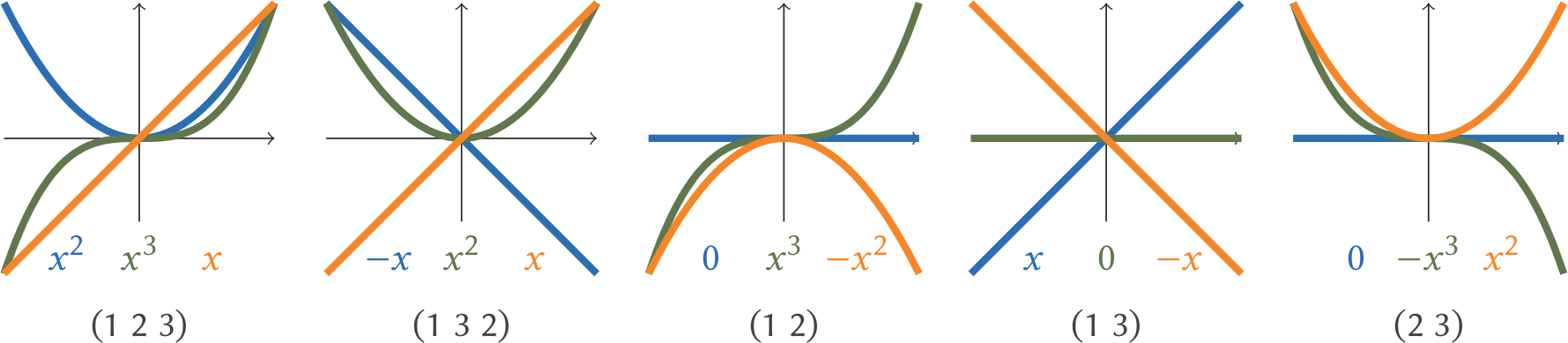## Possibilities with four polynomialsIn 2009, Maxim Konsevitch had shown the following: the permutations $(1\;3\;4\;2)$ and $(1\;2\;4\;3)$ cannot be realised by polynomials. All other 22 permutations of $\left\{ 1,2,3,4 \right\}$ can be realised, yet these 2 mysterious configurations are not possible to achieve with polynomial functions. To prove the permittable permutations is to write down 22 cases: an engaging activity of which it would be criminal to deprive the reader. To prove the impossibility of our mysterious pair, we will need to pull the concept of a valuation from our algebraic minibar.

Suppose $f(x)=a_n x^n +…+a_1 x + a_0$ is a real polynomial: a polynomial with real numbers for coefficients. The valuation of $f$ at $x=0$, $v(f)$, is the smallest $i$ with $a_i\neq 0$. For example,

$$v(8x^{2} + 20x)=1$$
and
$$v(x^{20} + 600 x^{10} – x)= 1.$$

Close to the origin, the smallest terms serve the most decisive role. The valuation of a constant is defined to be $\infty$.

If you have yourself been gathering the 22 polynomial cases, you have perhaps noticed that it is useful to treat the $x$-axis itself as a polynomial, simplifying the problem. (To achieve this, we may pick a polynomial $f_k$ to straighten out and replace all polynomials $f_i$ under consideration by $f_i – f_k$. For the purposes of our proof, pick $f_k$ to be $f_4$, one that’s the bottom of the bunch to the left of the axis.)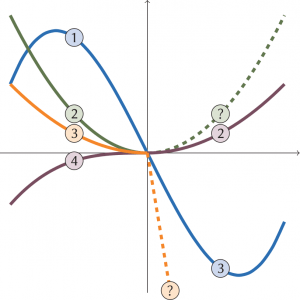$(1\; 3\; 4 \; 2)$ gives us trouble

Imagine the four polynomials ambling from left to right in time, crossing over the origin and switching places in the process in accordance with the target permutation $(1\;3\;4\;2)$. Under our assumption, to the left of the origin and before the mixing, $f_4$ is at the bottom (our newly minted $x$-axis) and the other 3 polynomials are above. Since $f_4$ goes to second place under $(1\;3\;4\;2)$, we must have 2 polynomials which were previously above $f_4$ for $x<0$ but now are below for $x>0$. Making use of our assumption that $f_4$ is the $x$-axis, we look for 2 polynomials (our $f_1$ and $f_3$) which cross the $x$-axis at the origin, with $f_1$, now in 3rd place, on top of the $f_3$, now fourth.

Crucially, we may observe that close to the origin, the non-constant polynomial with $v(f)=n$ is approximated by $a_n x^n$ : all other terms go to zero faster than the valuation’s term. But what of our valuation? It is useful to us precisely for the fact that $f(x)$ crosses the $x$-axis at the origin if and only if $v(f)$ is odd. And by definition it is the odd functions which have rotational symmetry and switch sign either side of the origin. Thus, near the origin, if $n$ is odd and $a_n$ is positive,

$$f(x) \approx a_n x^n \text{ which is }\begin{cases} >0 & \text{for }x<0,\\ <0 & \text{for }x>0. \end{cases}$$

What of it? We now have established $v(f_3)$ and $v(f_1)$ to be odd and $v(f_2)$ to be even. The parity is different. Let’s look at the order in which these polynomials appear more closely.

To the left of the origin,

$$f_1(x)>f_2(x)>f_3 (x) >0$$

by our choice of indices; to the right, $f_2(x)>0>f_1(x)>f_3(x).$ And near the origin, in the good company of fractions, each function looks much like its valuation-exponent power of $x$. Inching closer to $(0,0)$ as necessary, we find $v(f_1)\geq v(f_2)\geq v(f_3)$. We had glimpsed earlier that $f_1(x)>f_3(x)$ to the right of the origin, so $v(f_3) \geq v(f_1)$, as the 3rd function decreases faster that the 1st.

There is only one way to interpret $v(f_1)\geq v(f_2)\geq v(f_3) \geq v(f_1)$: equality all round! Yet in the last paragraph, we noted the parity must be different. This leads us to a contradiction.

But must we repeat the same song and dance for the $(1\;2\;4\;3)$ configuration? No: it is enough to note that this is the opposite cycle. Take the picture we painted for $(1\;3\;4\;2)$, hold it up to the mirror, and see the impossibility of $(1\;2\;4\;3)$!

## Back for more (polynomials)

What wonders lie beyond groups of four polynomials crossing at the origin? In his 2013 paper Intersecting Curves, Étienne Ghys showed that every permutation in $S_n$ is realisable—provided that the permutation did not ask any subset of the four polynomials to alternate in the unachievable $(1\;3\;4\;2)$ and $(1\;2\;4\;3)$ configurations. Make sure those 2 permutations are not in your entourage and the polynomials can enter from the left and emerge to the right of (0,0) in any order they please! But if you pick four of them and try to force the bottom of the group to be second, and the third to be the bottom, you’ll have an impossibility on your hands. You’ll be the butt of the joke!

It is easy to show necessity: erase all other polynomials but any four and we see that the forbidden permutations are as forbidden as ever. Yet is tricky to show this as a sufficient condition.

For this proof, we need graph theory: we must walk out of our pub at the origin and into a garden of pruned trees. We must build a tree for every collection of polynomials, starting from a constant-term-is-zero-for-all root, to linear terms and beyond, assigning a level to each degree and a node to each set of polynomials with identical terms of that degree. The leaves of such a tree will stand for the highest degree terms which help set all polynomials in a group apart. But alas, we must prune these trees if we have any hope that a computer may count the great many of them! Finally, by induction, we can describe, from a tree of $n-1$ polynomials, how to introduce the final $f_n$ and how we both can and cannot tack on an extra leaf. No $(1\;3\;4\;2)$ and $(1\;2\;4\;3)$ overlap will form.

Our tale ends here for now.